telescopeѲptics.net          ▪▪▪▪                                             CONTENTS

10.2.3.5. Maksutov-Cassegrain telescope off-axis aberrations

PAGE HIGHLIGHTS
Astigmatism   • Field curvature   • Example

Off-axis aberrations of the meniscus, unlike those of the Schmidt corrector, are not negligible. Both coma and astigmatism of the meniscus are of the opposite sign to those of the primary mirror, and of the same sign as those at the secondary. Due to the secondary spherical aberration contribution, also opposite to that of the primary (for spherical mirrors), the meniscus in two-mirror systems is somewhat weaker, thus also with somewhat lower contribution of (opposite) coma and astigmatism relative to those of the primary.

The primary coma aberration coefficient for the meniscus is, as mentioned, approximated by cL~-1.2q/ƒL2, q being the shape factor (R1+R2)/(R1-R2). With q expressed in terms of the meniscus focal length ƒL and first radius R1, it can also be written as cL= -1.2/R1ƒL. The system coma coefficient is a sum of the contributions from the lens and the two mirrors: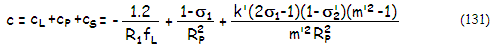also for spherical surfaces, with the values in the brackets, from left to right, being the aberration contributions of the meniscus lens cL, primary, cP, and secondary, cS, respectively. As before, σ1 is the stop (corrector) separation from the primary in units of the primary's r.o.c., k' is the relative height of marginal ray at the secondary, m' is secondary magnification and σ'2 the relative exit pupil separation for the secondary, obtained from Eq. 116.1 with σ1, k' and m' substituted for σ, k and m, respectively (m', k' and σ'2 represent the final values of these parameters determined by the corrector's power, as explained with Eq. 129). Substituting for ρ=mk/(m-1) in Eq. 116.1 and neglecting the prime notation for the parameter change due to the meniscus' power, gives approximation σ'2~{k-[1/(1-2σ1)]}(m-1)/2mk. Approximating σ1~(1-k)/2, which is nearly exact for the Gregory-Maksutov, gives σ'2~[k-(1/k)](m-1)/2mk.

The system P-V wavefront error is given by Wc=cαD3/12, with α being the field angle in radians. Expressing R1 and ƒL in terms of the primary's radius of curvature RP, the meniscus coma coefficient is approximated by cL~1.2t1/4/1.7RP9/4, t being, as before, the corrector center thickness. In terms of the coma of the primary mirror, for the typical relative separation σ1~0.4, that gives cL/cP~|t/RP|1/4. It shows that coma contribution of the corrector slowly increases with its relative thickness. However, this doesn't take into account the weaker corrector due to the presence of secondary mirror. With the secondary spherical aberration contribution approximated with -1.2k (from Eq. 154), in terms of the primary mirror contribution, and the aberration contribution of the corrector changing approximately in proportion to R14, the front meniscus radius needed to correct the two mirror's spherical aberration is weaker approximately by a factor of (1-1.2k)1/4. Since the meniscus' focal length ƒL changes nearly in proportion with R1, it gives better approximation of the corrector coma contribution as

cL/cP ~ 1.2|t/RP |1/4(1-1.2k)1/2.

For the average |t/RP|~0.02 ratio and k~0.25 for MCT with separated secondary, the corrector coma contribution is nearly -0.4 of the primary's (with the stop at the corrector), increasing only about 5% for k~0.2. For the secondary coma contribution, also in units of the primary's, substituting σ1~(1-k)/2 and σ'2~(m-1)/2mk2, gives it as approximately cS/cP~(m-1)/(1+k)m. For the average k~0.25 and m~5 (σ'2=-7.6), secondary's coma contribution is, from Eq. 131, about -0.63 of the primary's, exceeding -0.7 for k~0.2, m~6 and σ'2~-10. Since the secondary magnification m and relative minimum size k are inversely correlated as a function of the back focal length, for the typical MCT telescope the secondary coma contribution quite simply can be roughly approximated by cS/cP~-0.32/k.

The approximate coma contributions from the three MCT elements, as well as the resulting system coma, as a function of the relative meniscus thickness, are shown on FIG. 190.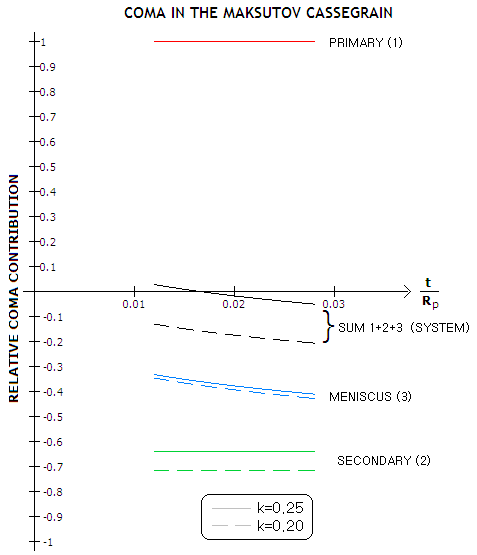FIGURE 190: Coma in the Maksutov Cassegrain is a sum of the coma contributions of the primary, secondary and meniscus. The secondary and meniscus induce coma of the same sign, and opposite to that of the primary, with the system coma being given by the sum of the three. For given meniscus thickness, the system coma nearly entirely depends on the minimum relative size of the secondary (k). Smaller secondary - hence generally higher secondary magnifications needed for a given back focus - increases the relative secondary contribution (in units of primary's contribution), as well as that of the meniscus, but only to a small extent for the latter. The meniscus' contribution increases more significantly with its relative thickness in units of the primary's radius of curvature (Rp). For the loose t/Rp average of ~0.02, the near-zero system coma is indicated for k slightly larger than 0.25 and the corresponding secondary magnification of approximately 4 to 5. Due to the limitation imposed by its secondary surface radius coinciding with the rear radius of the meniscus, the arrangement with a silvered spot on the meniscus (Gregory-Maksutov) requires higher secondary magnification - i.e. smaller minimum secondary size - resulting in the larger system coma. In general, these systems, if kept all spherical, cannot be coma-free, and the magnitude of coma, as indicated by the graph is around 15% of the primary's. Note that this is only for the lower-order (primary) coma. Secondary coma - originating mainly at the meniscus, negative in sign - can become significant in the systems with fast primaries. At ƒ/3 primary it is roughly 10% of the primary's coma in magnitude, and will add to the negative primary coma of the meniscus and secondary mirror.

The above indicates that the lower-order coma level in the MCT is generally low, as long as the usual designing freedoms are available. The coma is likely to be near zero for the ƒ/10-11 systems, while larger and  negative (tail down) with the slower ones, with the coma coefficient ranging from near-zero positive (~3% of the primary's, tail up) coma at ƒ/10 to nearly 15% of the primary's coma (opposite in sign, i.e. negative) at ƒ/15. With the P-V wavefront error given by Wc=cαD3/12, and the coma coefficient for a mirror with the stop at the surface given by cM=1/R2, becoming smaller by a factor 1-σ1 with displaced stop, σ1 being the stop (corrector) separation in units of primary's focal length, taking 150mm aperture, σ1=0.4 and ƒ/3 primary (so R=-900mm), the latter would come to 0.5 wave P-V (0.09 wave RMS) for 550nm wavelength at 0.5° off-axis for ƒ/15 system, and about 1/5 as much in an ƒ/10. In other words, lower-order coma is within diffraction limited over 0.4° field radius for the former, and about five times larger radius for the latter.

However, higher-order coma in the typical MCT is not negligible, and it combines with the lower order form in producing the final magnitude of the aberration. It is mainly produced by the corrector and negative in sign (tail down). This means that the two a smaller or greater degree offset one another when the lower-order form is positive, and add up when the lower order form is also negative. Going again from ƒ/10 to ƒ/15, the higher-order coma system coefficient increases by about 50%, from nearly twice the system's positive lower-order coma at ƒ/10 (about 5% of the nominal primary's lower-order coma) to about one half of the five times larger negative system coma at ƒ/15. Most of the increase is due to the stronger corrector needed to compensate for the lower spherical aberration contribution of the smaller secondary.

In other words, higher and lower-order coma at ƒ/10 partly offset one another, with the ~80% higher order excess, resulting in the predominantly higher-order form with nearly as large P-V wavefront error as the lower-order form alone, only of opposite sign. That would make the the combined higher and lower-order coma error in a 150mm ƒ/10 MCT at 0.5° off-axis is nearly 1/10 wave P-V. Or, for all practical purposes, non-existent (which is pretty much the case with the lower-order form alone).

Different story at ƒ/15. Here, the lower and higher order forms are of the same sign, and they are both larger than at ƒ/10, particularly the lower order form. The addition of the higher-order form increases the combined P-V wavefront error by about 50%, bringing it to nearly 3/4 wave P-V, or so, at 0.5° off-axis. However, the final error is is determined by the magnitude of astigmatism.

Lower-order astigmatism in the MCT is also generally low, but both, less predictable and potentially greater than either in the SCT or Houghton-Cassegrain. The reason is that neither Schmidt nor Houghton correctors have significant power, thus their astigmatic contribution is negligible. On the other hand, Maksutov corrector has relatively significant power and thickness, both resulting in a potentially significant amount of astigmatism, opposite in sign to that of the primary (hence of the same sign with the astigmatism of the secondary). So while the effect of the corrector's position on primary's astigmatism in a two-mirror system is generally similar to that in a Newtonian-style Maksutov or Schmidt, due to the stop (corrector) position relative to the primary being similar (typically, σ1~0.4), the system error is different. If astigmatism of the two mirrors nearly balances out, it leaves the meniscus' contribution as the dominant in the final system error. While it is relatively low, even low-level astigmatism can significantly change best field curvature, and also may become less than tolerable farther off-axis. If the secondary astigmatism overpowers that of the primary, combined with the meniscus' contribution it would likely result in a more than insignificant system error. And vice versa, if astigmatic contribution of the secondary is lower than that of the primary, astigmatism of the corrector will play balancing role.

As mentioned in the previous section, aberration coefficient of astigmatism for the Maksutov corrector is disproportionately greater than its power, due to it being a strongly curved thick meniscus qs illustrated at left.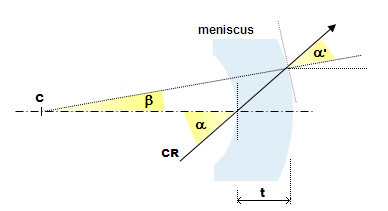Exaggeration of the effect of meniscus' thickness on its astigmatism (simplified; the actual CR path is refracted, involving meniscus' principal points, typically at a significant separation from the meniscus itself). While the effective focal length is relatively weak, both surfaces are strongly curved, of high powers and, consequently, astigmatism. As long as the meniscus is thin, their aberration nearly offset due to similar opposite powers. As the lens thickness (t) increases, the chief ray (CR) arriving at the front surface at an angle α, arrives at the second surface at an increasingly smaller angle α', due to an effective rotation of the second surface around its center of curvature C. The effective inclination angle α' at the second surface is given by α'=α. As a result, astigmatism at the second surface diminishes, which in turn increases the total lens' (meniscus) aberration. Depending on the system configuration, it may or may not be desirable.

While the aberration coefficient of astigmatism for a thin lens is a=-1/2ƒ, ƒ being the focal length, Maksutov corrector generates significantly more of the aberration, due to the imbalance in contributions of the two surfaces caused by meniscus' thickness (it also affects meniscus' coma, but to a lesser degree, due to it changing with the angle, not the square of it). Astigmatism added due to the meniscus' thickness, in a form of the aberration coefficient, is a'=t/2ƒ1R2, with ƒ1 being the first surface focal length. System coefficient of astigmatism is approximated by a~(n2+n-1)(n-1)t/2n2R1R2. Most of its numerical value comes from the portion caused by lens thickness, which can be written as a'=(n-1)t/2R1R2.

Thus the system aberration coefficient of astigmatism takes the form: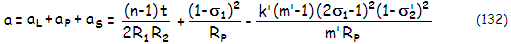with the system P-V wavefront error W=aα2D2/4 (note that σ2 is the stop separation for the secondary in units of its radius of curvature).

For n~1.5, the meniscus' coefficient can be approximated by aL~t/4R1R2, and taking R1~R2 gives aL~t/4R12. With R12~-|tRP3|1/2/3, the coefficient can be roughly approximated by aL~-3|t/RP|1/2/4RP which, for the typical average t/RP~0.02, gives the meniscus' astigmatism as 1/10 that of the primary's with the stop at its surface. Since mirror astigmatism changes with (1-σ1)2, for the typical corrector-to-mirror separation σ1~0.4RP, it gives corrector's astigmatism as aL/aP~-2|t/RP|1/2, in units of the primary mirror contribution. Again, as for the coma, this value needs to be adjusted for the weaker corrector due to the presence of the secondary mirror. The adjusted value is approximated by aL/aP~-2[|t/RP|(1-1.2k)]1/2. This puts corrector's astigmatism contribution to a system with the above parameters at nearly -1/4 that of the primary's.

For the average values of k~0.25, m~4 and σ1~0.4 (giving σ2'~-7.1), the secondary's astigmatic contribution is nearly -0.5/RP, or nearly 40% greater than that of the primary (~0.36/RP), and of the opposite sign. For smaller k and higher magnification m values, characteristic of Gregory-Maksutov in general, the relative secondary contribution can be considerably higher, mostly due to the increase in σ2' (Eq. 132/131). Roughly, astigmatic contribution in the typical Maksutov-Gregory is double that in usually somewhat faster MCT with a larger, separated secondary. Thus, the secondary contribution can be roughly approximated by aS/aP~-1/12k2, in units of the primary mirror astigmatic contribution.

Hence, the MCT system coefficient of astigmatism, as a sum of its elements' contributions, is approximated by a~{1-2[|t/RP|(1-1.2k)]1/2-(1/12k2)}(1-σ1)2/RP.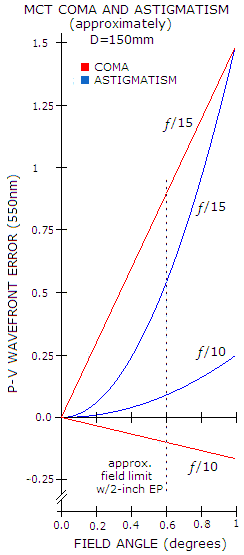In the actual, typical MCT configurations, similarly to coma, astigmatism is lower at ƒ/10 than at ƒ/15, mainly due to the smaller secondary inducing roughly twice more astigmatism. Both, meniscus and secondary induce astigmatism opposite to that of the mirror, the former roughly 1/4 to 1/3 as much as the primary (more at ƒ/15 than at ƒ/10), and the latter from about as much at ƒ/10 to nearly twice as much at ƒ/15 (both vs. primary's astigmatism with the stop at its surface). Hence the astigmatism aberration coefficient ranges from about 1/5 to about 6/5 of the mirror's (opposite in sign). With the mirror aberration coefficient being aM=1/R with the stop at its surface, reduced by a factor of (1-σ1)2 in an MCT, and with the P-V wavefront error given by W=aα2D2/4, taking 150mm aperture (so R=-900mm), σ1=0.4 and ƒ/3 primary gives the corresponding range of 1/16 to 1/2.7 wave P-V of astigmatism (0.013 to 0.074 waves RMS, for 550nm wavelength) at 0.5° off-axis, respectively. Higher-order astigmatism is negligible.

Thus, MCT lower-order astigmatism ranges from practically non-existent at ƒ/10 to low, visually inconsequential at ƒ/15. However, the level of astigmatism directly influences the best image field curvature. Typically, the combined astigmatism of the meniscus and secondary, which is of the same sign, is greater than astigmatism of the opposite sign induced by the primary. Thus the system astigmatism is opposite in sign to the system Petzval, hence making the best image surface less curved. However, with the astigmatism being relatively low in comparison to the Petzval, best image curvature remains generally strong (this concerns primary, or lower-order astigmatism; higher-order astigmatism is negligible).

Graph at left shows the approximate magnitude of coma (combined lower and higher order) and astigmatism for 150mm MCT, in the range from f/10 to f/15.

Image field curvature in the MCT is, similarly to other two-mirror arrangements, given in terms of the system's Petzval curvature R0 and aberration coefficient of astigmatism, as best, or median field curvature:Note that the meniscus doesn't affect appreciably system's Petzval curvature, which is for all practical purposes determined by mirror radii.

EXAMPLE: Actual vs. approximated level of coma and astigmatism in the 6" ƒ/3/17 Gregory-Maksutov and 6" ƒ/3/10 Maksutov-Cassegrain two-mirror system with a separate secondary from FIG. 119 (down-scaled to 6"). With t/RP being 0.021 for both, and k=0.18 and 0.27, respectively, the coma coefficient ratio approximation cL/cP~1.2|t/Rp|1/4(1-1.2k)1/2 gives the corrector lower-order coma contribution of about -0.4 and -0.37 in units of the primary's coma. Adding the secondary contribution of nearly -3/4 and about -0.6 of the primary's coma, respectively, according to cS/cP~-0.32/k, gives the approximated system lower-order coma sum of about -0.15 and 0.03 in units of the primary's coma for the Gregory-Maksutov and system with separate secondary, respectively.

The actual sums for the two systems are -0.19 and 0.03 of lower-order coma, respectively, with the higher order coma being nominally nearly 40% of the lower-order coma (thus adding up) in the former, and nearly identical, but of opposite sign (thus practically cancelling out) in the later. Approximations for lower-order coma are close enough to be useful.

Approximate values for the relative (in units of the primary's) astigmatism aberration contribution for the Maksutov-Gregory and the separate secondary MCT are, from aL/aP~-2[|t/RP|(1-1.2k)]1/2, -0.26 and -0.24 for the two correctors and, from aS/aP~-1/12k2, -2.5 and -1 for the secondary, respectively, in units of the primary's astigmatic contribution. The actual system contributions are -0.27/-0.22 and -2.3/-0.85, respectively. This puts the system astigmatism sum approximation at about -1.8 and -0.24, versus the actual -1.6 and -0.18, respectively. Again, not really accurate, but sufficiently so to reflect gross proportions of the individual elements' contribution and system error level.

Higher-order astigmatism in these systems is generally negligible.

From the above approximation for the system coefficient of astigmatism, it is a~0.0007 for the Maksutov-Gregory (slightly more than given with Eq. 132). With the system Petzval surface obtained from Eq. 30, best image surface curvature is, from Eq. 133, approximated as Rm~-190mm. The actual value for the system is -210mm, which is close enough for the initial assessment. For the system with separated secondary, the value of aL is only slightly (~10%) smaller, but the approximated astigmatism is nearly 1/4 of that of the primary, so that the system astigmatism coefficient (approximation) comes to a~0.00015. With that value, best (median) image curvature approximation for this system is Rm~-345mm, with the actual best field curvature Rm~-360mm.

The discrepancy is mainly caused by somewhat greater actual values for k, m and ρ than those obtained from two-mirror relations, the result of the power of meniscus corrector. For a quicker, and fairly accurate estimate of the median image curvature in an MCT, in a typical system it can be expected to be numerically quite close to the secondary mirror radius of curvature.